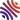## 46 publications1. Typed binary trees and generalized dendrifom algebras (), In J. Algebra, volume 586, . [hal-lmpa]
2. Chromatic polynomials and bialgebras of graphs (), In Int. Electron. J. Algebra, volume 30, . [hal-lmpa]
3. Algebraic structures on typed decorated rooted trees (), In SIGMA Symmetry Integrability Geom. Methods Appl., volume 17, . [hal-lmpa]
4. Free quadri-algebras and dual quadri-algebras (), In Comm. Algebra, volume 48, . [hal-lmpa]
5. Operads of (noncrossing) partitions, interacting bialgebras, and moment-cumulant relations ( and ), In Adv. Math., volume 369, . [hal-lmpa]
6. Lie theory for quasi-shuffle bialgebras (), Chapter in Periods in quantum field theory and arithmetic, Springer, Cham, volume 314, . [hal-lmpa]
7. Multigraded Dyson-Schwinger systems (), In J. Math. Phys., volume 61, .
8. Surjections as double posets (), In S'em. Lothar. Combin., volume 82, . [hal-lmpa]
9. Commutative and non-commutative bialgebras of quasi-posets and applications to Ehrhart polynomials (), In Adv. Pure Appl. Math., volume 10, . [hal-lmpa]
10. Extension of the product of a post-Lie algebra and application to the SISO feedback transformation group (), Chapter in Computation and combinatorics in dynamics, stochastics and control, Springer, Cham, volume 13, . [hal-lmpa]
11. Operads of finite posets ( and ), In Electron. J. Combin., volume 25, . [hal-lmpa]
12. A theory of pictures for quasi-posets ( and ), In J. Algebra, volume 477, . [hal-lmpa]
13. A divisibility result in combinatorics of generalized braids ( and ), In J. Combin. Theory Ser. A, volume 152, . [hal-lmpa]
14. The Hopf algebra of finite topologies and mould composition ( and ), In Ann. Inst. Fourier (Grenoble), volume 67, . [hal]
15. Deformations of shuffles and quasi-shuffles (, and ), In Ann. Inst. Fourier (Grenoble), volume 66, . [hal-lmpa]
16. Infinitesimal and $B_\infty$-algebras, finite spaces, and quasi-symmetric functions (, and ), In J. Pure Appl. Algebra, volume 220, . [hal-lmpa]
17. Pre-Lie algebras and systems of Dyson-Schwinger equations (), Chapter in Fa`a di Bruno Hopf algebras, Dyson-Schwinger equations, and Lie-Butcher series, Eur. Math. Soc., Z"urich, volume 21, .
18. A pre-Lie algebra associated to a linear endomorphism and related algebraic structures (), In Eur. J. Math., volume 1, .
19. The Hopf algebra of Fliess operators and its dual pre-Lie algebra (), In Comm. Algebra, volume 43, . [hal-lmpa]
20. The Hopf algebra of finite topologies and $T$-partitions ( and ), In J. Algebra, volume 438, . [hal-lmpa]
21. A combinatorial non-commutative Hopf algebra of graphs (, , , and ), In Discrete Math. Theor. Comput. Sci., volume 16, . [hal-lmpa]
22. Deformation of the Hopf algebra of plane posets (), In European J. Combin., volume 38, . [hal]
23. Polynomial realizations of some combinatorial Hopf algebras (, and ), In J. Noncommut. Geom., volume 8, . [hal]
24. Bruhat order on plane posets and applications (), In J. Combin. Theory Ser. A, volume 126, . [hal]
25. General Dyson-Schwinger equations and systems (), In Comm. Math. Phys., volume 327, . [hal]
26. Natural endomorphisms of shuffle algebras ( and ), In Internat. J. Algebra Comput., volume 23, . [hal]
27. Plane posets, special posets, and permutations (), In Adv. Math., volume 240, . [hal]
28. Ordered forests, permutations, and iterated integrals ( and ), In Int. Math. Res. Not. IMRN, . [hal]
29. Algebraic structures on double and plane posets (), In J. Algebraic Combin., volume 37, . [hal]
30. Free and cofree Hopf algebras (), In J. Pure Appl. Algebra, volume 216, . [hal]
31. Ordered forests and parking functions (), In Int. Math. Res. Not. IMRN, . [hal]
32. Lie algebras associated to systems of Dyson-Schwinger equations (), In Adv. Math., volume 226, . [hal-lmpa]
33. Primitive elements of the Hopf algebra of free quasi-symmetric functions (), Chapter in Combinatorics and physics, Amer. Math. Soc., Providence, RI, volume 539, . [hal-lmpa]
34. Hopf subalgebras of rooted trees from Dyson-Schwinger equations (), Chapter in Motives, quantum field theory, and pseudodifferential operators, Amer. Math. Soc., Providence, RI, volume 12, .
35. Free brace algebras are free pre-Lie algebras (), In Comm. Algebra, volume 38, . [hal]
36. Classification of systems of Dyson-Schwinger equations of the Hopf algebra of decorated rooted trees (), In Adv. Math., volume 224, . [hal-lmpa]
37. The infinitesimal Hopf algebra and the operads of planar forests (), In Int. Math. Res. Not. IMRN, . [hal]
38. The infinitesimal Hopf algebra and the poset of planar forests (), In J. Algebraic Combin., volume 30, . [hal]
39. Le groupe des traces de Poisson de certaines algèbres d'invariants ( and ), In Comm. Algebra, volume 37, . [hal]
40. Faà di Bruno subalgebras of the Hopf algebra of planar trees from combinatorial Dyson-Schwinger equations (), In Adv. Math., volume 218, . [hal]
41. Étude de l'algèbre de Lie double des arbres enracinés décorés (), In Adv. Math., volume 208, . [hal]
42. Bidendriform bialgebras, trees, and free quasi-symmetric functions (), In J. Pure Appl. Algebra, volume 209, . [hal]
43. Quantifications des algèbres de Hopf d'arbres plans décorés et lien avec les groupes quantiques (), In Bull. Sci. Math., volume 127, . [hal-lmpa]
44. Finite-dimensional comodules over the Hopf algebra of rooted trees (), In J. Algebra, volume 255, . [hal]
45. Les algèbres de Hopf des arbres enracinés décorés. II (), In Bull. Sci. Math., volume 126, . [hal-lmpa]
46. Les algèbres de Hopf des arbres enracinés décorés. I (), In Bull. Sci. Math., volume 126, . [hal-lmpa]